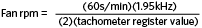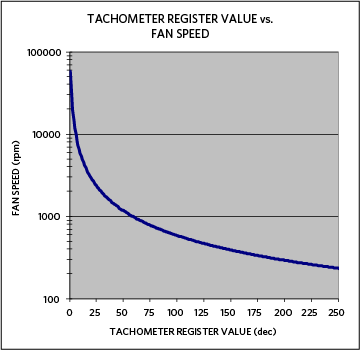# Calculating the Tachometer Register Value of the MAX6615/MAX6616

### Abstract

This application note explains how the MAX6615/MAX6616 temperature monitors and fan-speed controllers calculate the tachometer register value. The relationship between tachometer resolution and fan speed is also discussed.

### Calculating the Tachometer Count

To determine the value of the tachometer register for a given fan, you need to know several values: the internal clock frequency of the MAX6615/MAX6616 used for counting; the maximum rated rpm (revolutions per minute) of the fan; and the number of pulses that the fan issues per revolution.

The MAX6615/MAX6616 have an internal clock counter which runs at 1.95kHz. Every 67 seconds, the MAX6615/MAX6616 count the number of clock cycles that occur between two consecutive tachometer pulses, edge to edge, of the attached fan. The number of clock pulses is stored in the corresponding fan tachometer register.

When determining the tachometer value, first calculate the time between fan pulses. To do so, you must know the maximum rated fan speed (rpm) and the number of pulses issued per revolution for the chosen fan. The fan's rpm value is divided by 60 seconds per minute to get the rps (revolutions per second) value. That rps value is then multiplied by the number of pulses per revolution. The result is the number of pulses per second which the fan generates at maximum speed. If this value is inverted, then you determine the time between fan pulses.

As an example, if a 4000rpm fan produces two pulses per revolution, then the number of pulses per second would be 133.33. To determine the time between consecutive pulses, invert the value of 133.33 pulses per second and get 7.50ms/pulse.

Now that you know the time between pulse edges, you can determine how many clock cycles occur between the pulse edges. Since the MAX6615/MAX6616 use a 1.95kHz clock for the tachometer counter, you can determine the value of the tachometer register by multiplying the time between pulses by the clock-counter frequency of 1.95kHz.

Continuing the 4000rpm fan example, take the time between pulses, i.e., 7.50ms/pulse, and multiply that by the clock-counter frequency of 1.95kHz. The result is 14.63 clocks per pulse. Consequently, a tachometer value of 14d (0Eh) would be placed into the tachometer register for that fan. This will be the value for the maximum speed of that fan. If the fan were to reduce speed, there would be fewer revolutions per second; the value in the tachometer register would increase as the number of clock cycles per pulse would increase because of the greater time between pulses. Thus, the higher the value in the tachometer register, the lower the fan's speed.

### Determining Tachometer Register Resolution

To determine the resolution of the tachometer measurements, use the above steps to create an equation to represent the fan rpm-to-tachometer-count relationship. This relationship can be simplified to the following equation:Using this equation, you can input the possible values for the tachometer register (0 to 255 decimal) and determine the corresponding fan rpm value. Figure 1 shows the relationship between fan rpm and tachometer register value.Tachometer measurement resolution is best when the fan rpm value is 4000rpm or less for a two-pulse-per-revolution fan. The maximum fan speed that the MAX6615/MAX6616 can measure for a two-pulse-per-revolution fan is 58.5krpm, as that corresponds to a tachometer register value of 01h. This extended range is very useful when reading the tachometer register to determine if the attached fan is running.